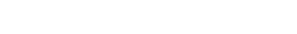# SIER Working Paper Series

## 128 Factor-Driven Two-Regime Regression

• Sokbae Lee, Yuan Liao, Myung Hwan Seo, Youngki Shin
• 128.pdf

#### Abstract

We propose a novel two-regime regression model where the switching between the regimes is driven by a vector of possibly unobservable factors. When the factors are latent, we estimate them by the principal component analysis of a panel data set. We show that the optimization problem can be reformulated as mixed integer optimization and present two alternative computational algorithms. We derive the asymptotic distributions of the resulting estimators under the scheme that the threshold effect shrinks to zero. In particular, we establish a phase transition that describes the effect of first stage factor estimation as the cross-sectional dimension of panel data increases relative to the time-series dimension. Moreover, we develop a consistent factor selection procedure with a penalty term on the number of factors and present bootstrap methods for carrying out inference and testing linearity with the aid of efficient computational algorithms. Finally, we illustrate our methods via numerical studies.
Keywords: threshold regression, mixed integer optimization, phase transition, oracle properties, L0-penalization